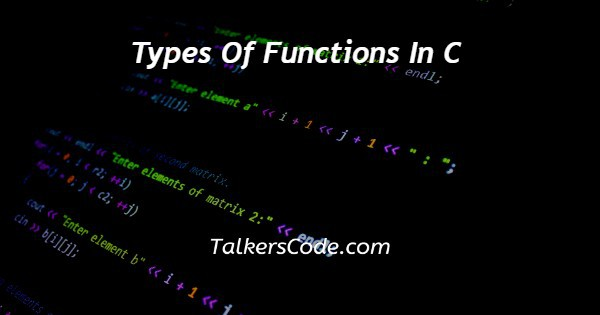# Types Of Functions In C

Last Updated : Jan 1, 2023In this article we will show you the solution of types of functions in C, firstly we understand that what is type and function. In simple terms we can say that type is a kind or it is a variety or classification.

Function means the task or any action to achieve something. Now, let us understand the concept Function Types in C Language

## Step By Step Guide On Types Of Functions In C :-

### Following are the types of functions in C Language -

Standard Library Functions - Also referred to as predefined functions, library functions are formerly defined in the C libraries. This means that we don’t have to write a description or the function’s body to call them.

We can simply call them without defining them as they’re formerly defined. Still, we need to include the library at the morning of the law for calling a library function. We can also use the proper syntax of the function to call them. Printf(), scanf(), ceil(), and floor() are samples of library functions.

User-Defined Functions - The functions which are declared, defines and calls by developer or user in a program.'

This increases the range and functionality, and reusability of C programming as we can define and apply any function we require.

A considerable plus point of C programming is that we can append a user- defined to any library to use it in distinct programs.

Let us understand standard library function in following code

```#include <stdio.h>
int main() {
printf("Talkerscode!!");
return 0;
}```
1. In first line of the code we use pre-processor directory and header file i.e. stdio.h it is the standard library
2. Then we use return_type as a int and after that our main function begins.
3. Then you can see that our function body in which we write the statement which we have to print in our output by using printf.
4. At the end of code we write return 0 it means or program executed successfully.
```#include<stdio.h>
int sum(int a, int b)
{
return a+b;
}
int main()
{
int ans;
ans=sum(11,3);
printf("%d",ans);
return 0;
}```
1. In first line of the code we use pre-processor directory and header file i.e. stdio.h it is the standard library
2. Then we create user-defined function named as int sum.
3. After that creating function we passes parameters in it.
4. Then we call the function.
5. Then you can see that our function body in which we write the statement which we have to print in our output by using printf.
6. At the end of code we write return 0 it means or program executed successfully.

## Conclusion :-

Hence, we have successfully learnt the types of functions in C language we also learnt that how to create user defined functions and how to use standard library functions.

I hope this article on types of functions in C helps you and the steps and method mentioned above are easy to follow and implement.